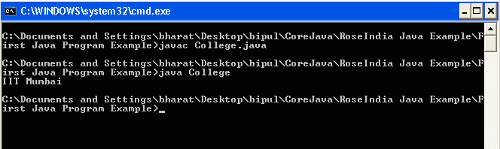Tutorials

# First Java Program Example

In this section we will discuss about the first java program example.

In this section we will discuss about the first java program example.

## First Java Program Example

In this section we will discuss about the first java program example.

To create a Java program we would be required to create a Java class. In this class we will print a string like "IIT Mumbai". To demonstrate about a Java program I am giving a simple example.

Example

Here I am giving a simple example which will demonstrate you about how to write your first program in Java. In this example I have created a simple class named College.java. In side this class created a method named displayDetails() which will print the string "IIT Mumbai" on call. This class also contained a public static main method inside which Instantiate the class and called the method displayDetails().

Source Code

```public class College
{
String str = "IIT Mumbai";

public void displayDetails()
{
System.out.println(str);
}
public static void main(String args[])
{
College college = new College();
college.displayDetails();
}
}
```

Output